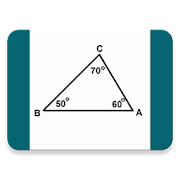## Angle Calculator helps you practice Grade HS Math - Lumos Educational App StorePrice -fREE

#### DESCRIPTION:

Angle Calculator is simple app that visualize the calculation result of angles from triangles, square, pentagon, hexagon and many more. You can interact with drag the point to find the angles that you want calculated. Let try and have fun!

#### OVERVIEW:

Angle Calculator is a free educational mobile app By .It helps students in grades HS practice the following standards HSG.CO.C.10.

This page not only allows students and teachers download Angle Calculator but also find engaging Sample Questions, Videos, Pins, Worksheets, Books related to the following topics.

1. HSG.CO.C.10 : Prove theorems about triangles. Theorems include: measures of interior angles of a triangle sum to 180°; base angles of isosceles triangles are congruent; the segment joining midpoints of two sides of a triangle is parallel to the third side and half the length; the medians of a triangle meet at a point..

HS

#### STANDARDS:

HSG.CO.C.10

Software Version: 1.0.3

Category:

### RELATED APPS

Rate this App?
0

0 Ratings & 0 Reviews

5
0
0
4
0
0
3
0
0
2
0
0
1
0
0EdSearch WebSearch